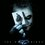# Some Properties.

(1) Reflexive Property-- A quantity is congruent (equal) to itself. $a=a$

(2) Symmetric Property-- If $a = b$, then $b = a$.

(3) Transitive Property-- If $a = b$ and $b = c$, then $a = c$.

(4) Addition Postulate-- If equal quantities are added to equal quantities, the sums are equal.

(5) Subtraction Postulate-- If equal quantities are subtracted from equal quantities, the differences are equal.

(6) Multiplication Postulate-- If equal quantities are multiplied by equal quantities, the products are equal. (also Doubles of equal quantities are equal.)

(7) Division Postulate-- If equal quantities are divided by equal nonzero quantities, the quotients are equal. (also Halves of equal quantities are equal.)

(8) Substitution Postulate-- A quantity may be substituted for its equal in any expression.

(9) Partition Postulate-- The whole is equal to the sum of its parts.

Also: Betweeness of Points: $AB + BC = AC$.

Angle Addition Postulate: $\angle ABC + \angle CBD = \angle ABD$.

(10) Construction-- Two points determine a straight line.

(11) Construction-- From a given point on (or not on) a line, one and only one perpendicular can be drawn to the line.Note by Sattik Biswas
5 years, 2 months ago

This discussion board is a place to discuss our Daily Challenges and the math and science related to those challenges. Explanations are more than just a solution — they should explain the steps and thinking strategies that you used to obtain the solution. Comments should further the discussion of math and science.

When posting on Brilliant:

• Use the emojis to react to an explanation, whether you're congratulating a job well done , or just really confused .
• Ask specific questions about the challenge or the steps in somebody's explanation. Well-posed questions can add a lot to the discussion, but posting "I don't understand!" doesn't help anyone.
• Try to contribute something new to the discussion, whether it is an extension, generalization or other idea related to the challenge.
• Stay on topic — we're all here to learn more about math and science, not to hear about your favorite get-rich-quick scheme or current world events.

MarkdownAppears as
*italics* or _italics_ italics
**bold** or __bold__ bold
- bulleted- list
• bulleted
• list
1. numbered2. list
1. numbered
2. list
Note: you must add a full line of space before and after lists for them to show up correctly
paragraph 1paragraph 2

paragraph 1

paragraph 2

[example link](https://brilliant.org)example link
> This is a quote
This is a quote
    # I indented these lines
# 4 spaces, and now they show
# up as a code block.

print "hello world"
# I indented these lines
# 4 spaces, and now they show
# up as a code block.

print "hello world"
MathAppears as
Remember to wrap math in $$ ... $$ or $ ... $ to ensure proper formatting.
2 \times 3 $2 \times 3$
2^{34} $2^{34}$
a_{i-1} $a_{i-1}$
\frac{2}{3} $\frac{2}{3}$
\sqrt{2} $\sqrt{2}$
\sum_{i=1}^3 $\sum_{i=1}^3$
\sin \theta $\sin \theta$
\boxed{123} $\boxed{123}$

## Comments

Sort by:

Top Newest

You are really diverse, i must say. From reflexive and symmetric to primitive roots. Wow!

- 5 years, 2 months ago

Log in to reply

hahaha yup I am..

- 5 years, 2 months ago

Log in to reply

I just like to explore the world.

- 5 years, 2 months ago

Log in to reply

This is interesting. Why don't you add a small introduction at the top?

As a moderator, I am very appreciative of your notes. Since you are into this, I suggest you read what other people share too for inspiration. You'll find some intriguing examples at the bottom of this page

- 5 years, 2 months ago

Log in to reply

Also, you could contribute your knowledge in the wikis

Several of them need examples, feedback and completion. You could also start your own wiki. You could let the world know about them by maintaining a note like this or sharing the wiki links on social media

- 5 years, 2 months ago

Log in to reply

Okay thanks!

- 5 years, 2 months ago

Log in to reply

×

Problem Loading...

Note Loading...

Set Loading...